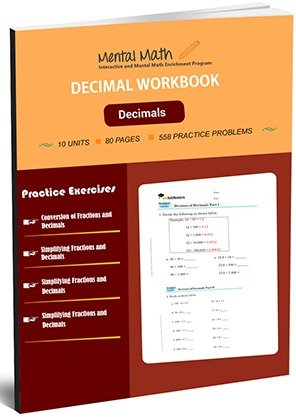20,864

# Decimals Worksheets\$7.00

A decimal is a fraction having denominator as the power of 10. So, students start decimal concept with converting fractions into decimals and vice-versa. Students practice more problems related to four operations on fractions and decimals than previous fraction concept. Then, they will get knowledge of adding, subtracting, multiplying and dividing decimals in this section.

Worksheet # Topics/Curriculum # of Pages Sample Add to cart
Unit-1 Conversion of Fractions and Decimals 8 pages\$1.00

Unit-2 Fractions and Decimals Operation 8 pages\$1.00

Unit-3 Simplifying Fractions and Decimals 8 pages\$1.00

Unit-4 More Problems on Simplifying Fractions and Decimals 8 pages\$1.00

Unit-5 Four Operations on Fractions and Decimals 8 pages\$1.00

Unit-6 More Problems on Four Operations on Fractions and Decimals 8 pages\$1.00

Unit-7 Addition of Decimals 8 pages\$1.00

Unit-8 Subtraction of Decimals 8 pages\$1.00

Unit-9 Multiplication of Decimals 8 pages\$1.00

Unit-10 Division of Decimals 8 pages\$1.00

## Choose From Over 15 Worksheets That Best Suit Your Child’s Skill Level and Unique Needs

You can purchase our high quality math worksheets separately, or purchase an entire book and save 40%!

### Equation Worksheets

Evaluate Algebraic Expressions and Solve Equations

See all units

### Integers Worksheets

Four Operations on integers and their Simplification

See all units

### Decimals Worksheets

Decimal Concept and Four Operations on Decimals

See all units

### Fractions Worksheets

Fraction Concepts and Four Operations on Fractions

See all units

### Division Worksheets

Dividing up to 4 or more digit Numbers by 2 or 3-digit Numbers

See all units

### Multiplication Worksheets

Multiplication Table of 2 through 10 and Multiplication by Thousands

See all units

### Subtraction Worksheets

Subtracting 4 to 1-digit Numbers form 4 digit Numbers

See all units